# What Is Mental Math? Strategies & Examples

You might be wondering, "What is mental math, why is it important for kids or what is an example of mental math?" Mental math refers to the practice of performing calculations and solving mathematical problems using only mental processes, without the use of pencil and paper.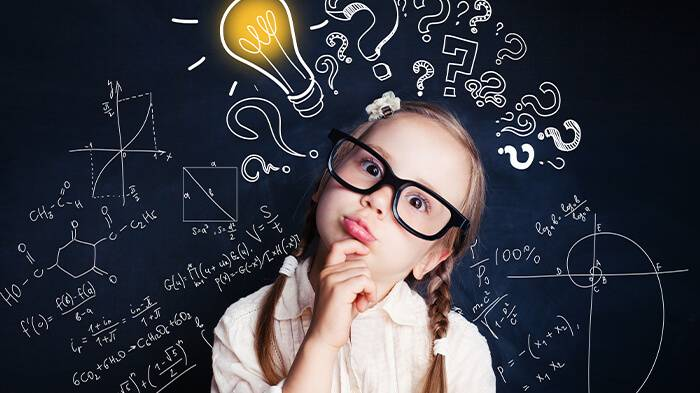It enhances kids' number sense, improves their problem-solving skills, and boosts their confidence in handling everyday mathematical situations. In this blog post, we will explain how you can enhance your kid's mental math skills and share some of the best examples.

## What Is a Mental Math Strategy? The Best Methods to Improve

If you want to know what type of skill is mental math, we can say that it is a skill that kids can improve over time. Here are some of the best methods to develop mental math skills.

### 1. Playing MentalUP Educational Games

MentalUP's games captivate young minds with an array of mathematical challenges, puzzles, and tasks. Kids adore, and parents rely on MentalUP for boosting mental math skills.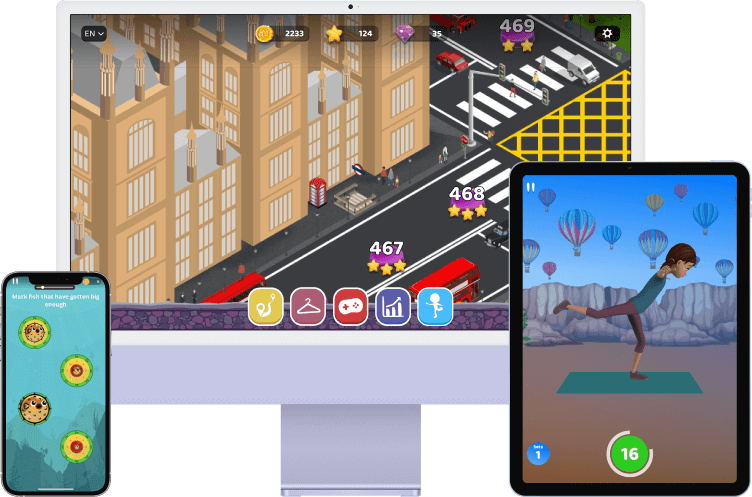MentalUP is a scientifically crafted learning app that offers hundreds of personalized cognitive development games and activities that seamlessly blend entertainment and education, fostering skills like problem-solving, critical thinking, and swift mental calculations.

Consistent engagement with these games cultivates improved memory, heightened attention, and enhanced cognitive math-related abilities. Plus, the app can be used on any PC, tablet, or smartphone to nurture kids' minds anytime and anywhere!

Looking for a proven way to foster your child’s math skills? Look no further!

MentalUP is an Evidence of Impact awarded learning app that caters to kids of all ages and even adults. Thanks to its engaging platform, you can access 150+ mental math activities that’ll keep all your family members' mental skills active. 🙌

MentalUP has no ads, meaning that it is one of the safest and most effective resources for both yourself and your kids. Join the millions of parents and kids who have enjoyed taking their mental skills to the next level with MentalUP! 👪

### 2. Using Calculators Less Often

If you think, “What is the skill of doing mental math, we can say that it is definitely related to reducing reliance on calculators. Relying less on calculators forces kids' brains to engage in mathematical calculations more frequently. This practice helps in strengthening their mental math skills over time.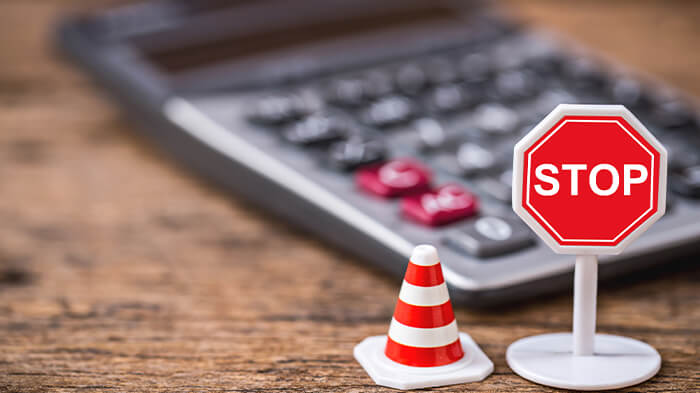So, you can encourage your kids to use calculators less often and let them calculate small problems mentally. This method might seem hard at the beginning, but it will promote a better number sense and understanding of mathematical concepts for your kids.

### 3. Chunking Calculations

If you are wondering, “What is mental math strategies that can be highly beneficial for kids?”, you can try teaching them to chunk complex calculations into smaller parts. This method makes math calculations less daunting and easier to handle.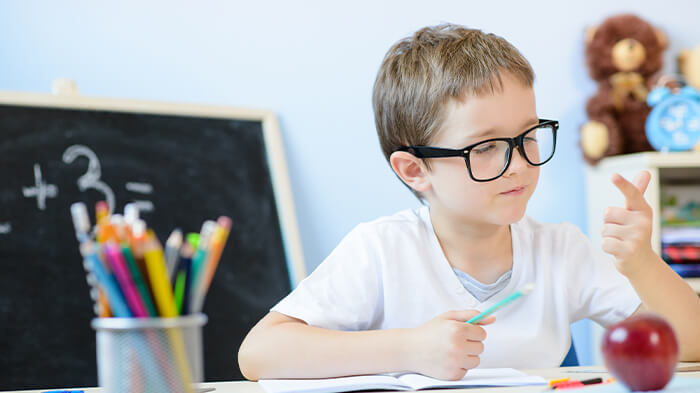By focusing on smaller pieces of the problem, kids can perform calculations more efficiently and accurately. It also helps reduce cognitive load, as kids' brains deal with fewer elements at a time, leading to better overall performance in math.

### 4. Practicing Estimation

Estimation means getting a close idea of the answer to math problems instead of trying to be exactly right. When kids practice estimation often, it helps them understand numbers better and quickly figure out if their answers make sense.This method is particularly beneficial for helping kids reinforce their familiarity with number magnitudes and relationships, which aids in more accurate mental calculations.

### 5. Familiarizing with Number Patterns

Number patterns are sequences of numbers that follow a certain rule or logic. Familiarizing with number patterns can help kids improve their mental math skills by recognizing and applying the rules of patterns to different situations.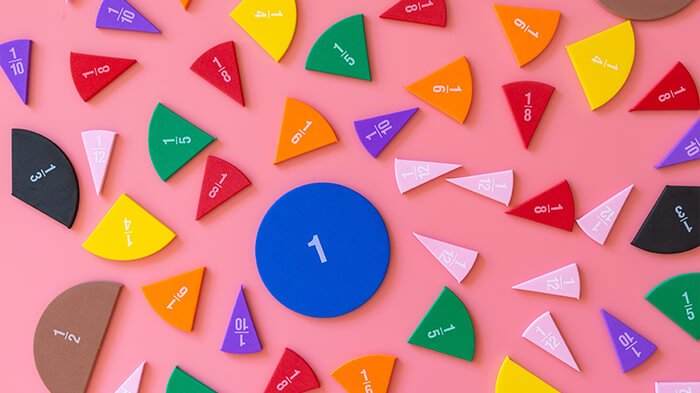For example, If a child knows the pattern of adding 2, they can easily find the next term, the nth term, or the sum of a certain number of terms in the sequence. This method also helps kids develop their algebraic thinking and spatial reasoning skills.

### 6. Practicing Regularly

If you are wondering, “What is a mental math strategy that’ll definitely help kids enhance their mental calculation abilities?” we can say that it starts with regular practice. This can help kids improve their mental math skills by strengthening their memory, concentration, and accuracy.It is recommended that kids engage in mental math exercises on a daily or weekly basis. This consistent practice will help children build their confidence and motivation in learning math, as they can track their progress and achievements over time.

## What Are Mental Math Examples? Improving Your Mental Calculations

Now that we’ve defined what is mental math mean, let's take a closer look at examples and techniques of mental math that are helpful for kids of all ages.

### 7. Counting On and Back

Wondering what is mental math 2nd grade kids can apply? Well, counting on and back is one of the easiest ones that all kids can utilize. This example involves adding or subtracting numbers by counting on or back from one of the numbers.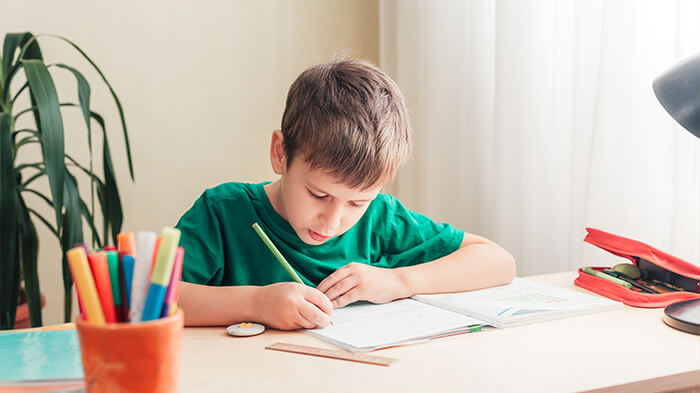For example, to add 7 and 4, you can start from 7 and count on four more numbers: 8, 9, 10, and 11. To subtract 7 from 11, you can start from 11 and count back seven numbers: 10, 9, 8, 7, 6, 5, and 4. This will help your kid develop their number sense and mental arithmetic skills.

Do you need a trustworthy resource to track your kids' development? MentalUP is here to help! 👏

In addition to its effective mental development and physical education activities, MentalUP offers robust performance-tracking and reporting tools to assist parents and teachers in keeping track of their kids' development. 📊

Let your kids embark on an exciting arithmetic journey where addition, subtraction, multiplication, and division become thrilling quests with MentalUP! ✨

### 8. Rounding Up to the Nearest Ten

One way to make addition and subtraction easier is to work with multiples of 10 because they don’t require borrowing or carrying. To do this, kids can round the numbers in a problem to the nearest 10, and then adjust the answer by adding or subtracting the difference.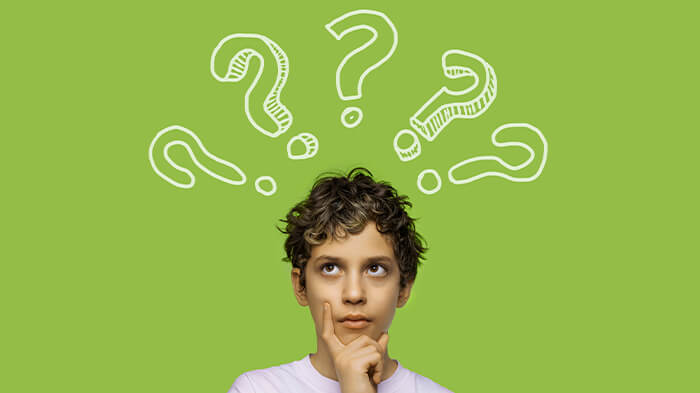For example, to add 37 and 25, you can round them to the nearest 10 by adding 40 and 30 to get 70. However, this is not the exact answer because you added 3 more to 37 and 5 more to 25. So you need to subtract the extra amount from 70: 70 - (3 + 5) = 70 - 8 = 62.

### 9. Working from Left to Right

If you’ve asked yourself, “What is mental math 6th grade kids can utilize?” you can explore working from left to right. It is a strategy that involves adding or subtracting numbers from left to right instead of from right to left as in the traditional algorithm.For example, to add 456 and 789, you can start by adding 400 and 700 to get 1100 then add 50 and 80 to get 130 then add six and nine to get fifteen. Finally combine the results to get:1100 +130 +15 =1245.

### 10. Using Multiplication Hacks

Using multiplication hacks is one of the best mental math methods to help kids develop a better understanding of place value. Once they learn the shortcuts, the multiplication process becomes much easier for kids.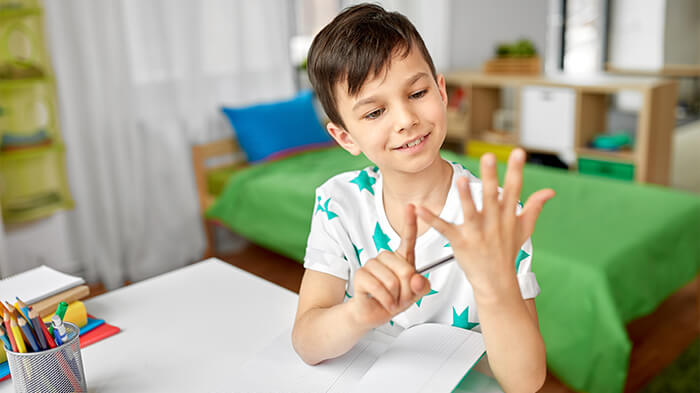They just need to keep in mind some rules. For example, any number multiplied by 1 stays the same. Any number multiplied by 10 has a zero added to its end. Any number up to 9 multiplied by 11 is just the same digit repeated twice (e.g. 8 x 11 = 88).

### 11. Bumping the Decimal Over

This method involves moving the decimal point over one place to the left or right to divide or multiply by 10, respectively. It is a mental math method for helping kids gain speed when calculating and learning fundamental mathematical concepts more profoundly.Let's understand this mental math method with an example: to divide 45 by 10, kids can shift the decimal over one place to the left and get 4.5. Similarly, to multiply 4.5 by 10, they can shift the decimal over one place to the right and get 45.

### 12. Near Doubles

If you are wondering, “What is mental math multiplication methods for kids of all ages?” you can check out this technique. The basic idea is to take advantage of two numbers that follow each other. By taking advantage of this closeness, kids can streamline their calculations.For example, let's take 5 + 6. By recognizing their consecutive relationship, you can break it down into 5 + 5 + 1, which equals 11. This method not only enhances math skills but also nurtures a deeper number sense in kids.

### 13. Multiplying 5 Times Any Number

If you’ve googled, “What are mental math examples?”, you’ve probably seen multiplying 5 times any number. This multiplication method works every time because multiplying by 5 is the same as multiplying by 10 and then dividing by 2.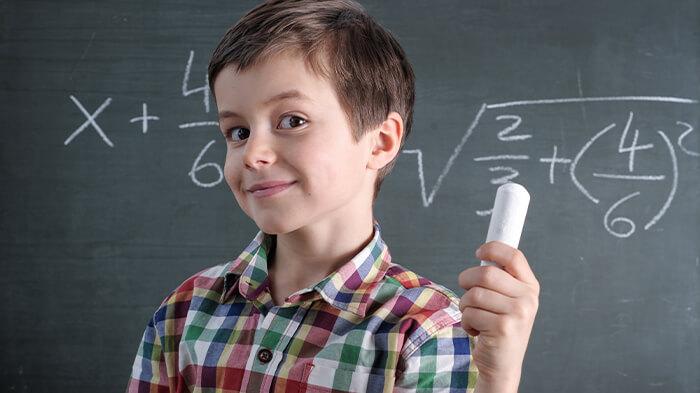When multiplying a number by 5, you can use a shortcut by simply dividing the number by 2 and then appending a zero to the result. For example, if you want to multiply 32 by 5, you can divide 32 by 2 to get 16, and then append a zero to get 160.

### 14. Multiplying Numbers that End in Zero

This is one of the most effective mental math methods for multiplication problems. When multiplying numbers that end in zero, kids can simplify the calculation by initially disregarding the zero(s), performing the multiplication, and then adding the appropriate number of zeros to the result.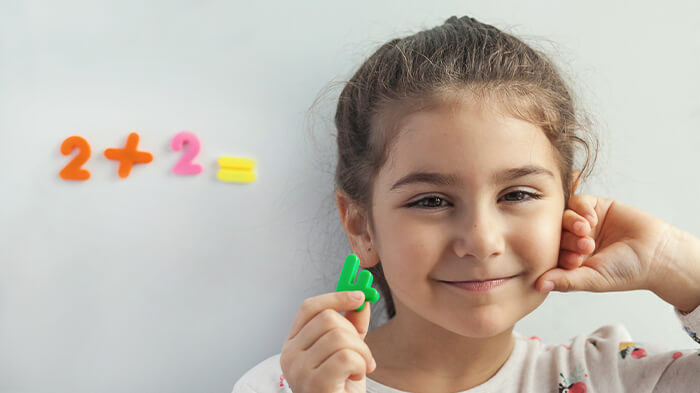For example, let's consider multiplying 320 by 40. First, ignore the zeros and multiply 32 by 4 to get 128. Then, append two zeros to the result, yielding the final answer: 12,800. This technique is particularly useful to help kids calculate faster.

## Simple Tips to Implement Mental Math & Improve Math Skills

Now that we've learned the most effective methods and examples for improving mental math skills let's see how we can incorporate mental math into our lives to enhance our mathematical abilities.

• Use mental math tricks in your daily life.
• Play math-related games that encourage quick thinking.
• Encourage estimation during activities, like measuring distances.
• Use visual aids to help children grasp mathematical concepts.
• Share interesting math facts or stories to spark curiosity in the subject.
• Create a supportive and positive environment for math learning.
• Celebrate small achievements in mental math skills.
• Offer challenges that gradually increase in difficulty.
• Teach mental math shortcuts and techniques.
• Present mental math tricks as fun puzzles to solve.
• Engage in discussions about how math is used in everyday life.
• Provide access to online math apps that offer engaging math practice.
• Model a positive attitude towards math and learning.
• Offer assistance when needed, but also encourage independent thinking.

Test, train, and improve your kid’s math skills with MentalUP! Everything you need to support their mathematical development is in MentalUP. 🚀

Kids of all ages will find effective and engaging math activities tailored for them. So why wait? You can start using the app right now to give your kid the gift of learning with MentalUP. 🎁

If “What is mental math?” is not the only question in your mind, here are the most commonly asked questions about the topic, along with their answers.

### How can I help my child with math at home?

Engage your child in real-life math situations, use everyday activities to demonstrate math concepts, and encourage problem-solving discussions. Additionally, provide supportive resources, like math games and online tutorials, to reinforce learning.

### What is the most effective way to practice mental math?

You can try regularly challenging your and your kid's mind with mental math exercises. Ensure that you begin with simple calculations and gradually increase complexity.

### Are there any applications or online tools I can use to hone my mental math abilities?

Yes, there are various apps, like MentalUP, that offer interactive practice for improving mental math skills.

### What is the importance of mental math?

Mental math enhances number sense, promotes quick thinking, aids in everyday problem solving, and builds confidence in mathematical abilities by reducing reliance on calculators.

### Can practicing mental math strengthen my memory and focus?

Yes, practicing mental math exercises can improve memory retention, boost cognitive focus, and sharpen overall mental agility.

### Can mental calculation tips improve the grades of students who struggle with math?

Yes, teaching mental calculation techniques can empower struggling students by providing alternative approaches to math problems, potentially leading to better understanding and improved grades.

### What types of games can teach kids how to get better at mental math?

Games like Math Bingo, 24 Game, and Math Jeopardy encourage mental math skills through exciting challenges, fostering a positive learning environment.

### How can I implement mental math in the classroom?

Incorporate mental math routines at the start of lessons, encourage peer-to-peer problem-solving discussions, and use interactive activities that promote mental calculation skills.

### Will these mental tricks positively impact performance at school?

Yes, mastering mental math techniques can boost problem-solving abilities, increase confidence in math-related tasks, and potentially lead to improved overall academic performance.

### Will mental calculation be tough for younger kids?

Mental calculation can be gradually introduced to younger children through playful activities and visual aids, helping them develop a strong foundation for more advanced skills.

### Why do we need to teach mental math and number facts?

Teaching mental math and number facts is crucial as it fosters number fluency, aids in mental flexibility, and equips individuals with essential skills for quick, accurate calculations in everyday situations and more advanced math concepts.

Long story short if you want to support your kids' mental math skills or even improve your own what you need is regular practice with effective and trustworthy resources.

Discover the ultimate app to keep mental and physical skills sharp - it's MentalUP! This scientifically designed learning app includes 150+ cognitive games and 240+ fitness exercises catering to a variety of age groups from kids to adults. 🏆

With MentalUP you can enhance the skills of all your family members through personalized 7-minute daily brain training and 20-minute daily exercise routines. It is time to unlock the potential of both your kids and yourself! 🔥

YOU MIGHT ALSO LIKE:

#### 15 Best Math Apps for Kids (Teacher-Recommended)

HOME PAGE / BLOG / What Is Mental Math? Strategies & Examples